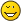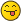Actual formulas for tetration and its derivativehyper4geekJunior FellowPosts: 3 Threads: 1 Joined: Aug 2007 08/31/2007, 03:10 PM Hello, I have played around with the concept of tetration for probably 10 years now and never really achieved much in that field. I studied Andrew Robbins paper quite a while ago and played around with numerical functions constructed from the values he computed, to finally create a HyperLog function (hyper-logarithm to base E) and a HyperExp function (hyperexponential function to base E) in the full complex plane. Now I always had some, let's call it "feelings" about what properties such functions had to have, tested that numerically and was stunned that it came out to be true using that numerical approximations. Here are my most valuable formulas regarding tetration. I don't know whether that new or not, so I post it here anyway. First of all, a little notation, because I don't use what's probably around. The following will be in a Mathematica-remniscent code. Let's define functions: E = euler's constant e Exp[x] = E*E*E*...*E (x times) TetraExp[x] = E^E^E^...^E (x times) TetraExpPrime[x] = first derivative of TetraExp[x] ProductLog[x] = lamberts W function = u in v==u*Exp[u] Here are formulas that define TetraExp using neighbors of TetraExpPrime: TetraExp[x] == TetraExpPrime[x] / TetraExpPrime[x-1] TetraExp[x] == ProductLog[TetraExpPrime[x+1] / TetraExpPrime[x-1]] And here are recurrence relations of TetraExpPrime: TetraExpPrime[x] == TetraExpPrime[x-1] * Exp[TetraExpPrime[x-1]/TetraExpPrime[x-2]] TetraExpPrime[x] == TetraExpPrime[x-1] * ProductLog[TetraExpPrime[x+1]/TetraExpPrime[x-1]] TetraExpPrime[x] == TetraExpPrime[x+1] / Log[TetraExpPrime[x+2]/TetraExpPrime[x+1]] Of course I don't have any means of proving that. Any comments still appreciated.bo198214Administrator Posts: 1,624 Threads: 103 Joined: Aug 2007 08/31/2007, 03:37 PM Wow, interesting (and hello and welcome on board!) However I didnt ever see those formulas. How did you verify them? Numerically computing the inverse of Andrew's slog and then numerically computing the derivatives? I think Jay is closest with his research, he computed several derivatives of the super exponential (derived from slog) up to a quite high precision. Perhaps he can just jump in, numerically verify the formulas and say something about themhyper4geekJunior FellowPosts: 3 Threads: 1 Joined: Aug 2007 08/31/2007, 05:05 PM (This post was last modified: 08/31/2007, 05:07 PM by hyper4geek.) bo198214 Wrote:How did you verify them? Numerically computing the inverse of Andrew's slog and then numerically computing the derivatives?That's exactly how I did it. The formulas are even valid for complex values of x. I used a series expansion derived from Andrew's formulas and computed them also in the complex plane. Despite of the radius of convergence, using some known recurrence relations, you can make the function convergent for almost any point in the complex plane. I just have still difficulties in the region very roughly around +/- I (imaginary i), where it doesn't converge regardless of what relations I use. It seems that at these points we have branch points and actually I found two distinct ways of placing branch cuts around these points. See the three images about TetraLog for this. The first two are centered at the origin and toward the left/right approach -Infinity/Infinity and toward the top/bottom I*Infinity / -I*Infinity. You can see the branch cuts along roughly I to Infinity and -I to -Infinity. An alternative placement of branch cuts is seen in the third image, which repeats its branch cut every 2 I Pi k (not seen in the image though). This image runs from -4 to 4 on the real axis (x) and from -4*I to 4*I on the imaginary axis (y). That TetraLog (slog) function is then easily (well numerically) invertible to give (what I call) TetraExp, however, because of the non-convergent TetraLog (slog) for the two regions I mentioned, I can only generalize the TetraExp function for complex values up to (real)x +/- 1 I. See the two images about TetraExp for this. They run from -4 to 4 on the real axis (x) and -1 I to I on the imaginary axis (y). Of course the images don't explain why of if the formulas are valid, but you can see that I had a lot of values to try out in the complex plane, and for every single point, the formulas were true even regardless of what branch cut definition I used for TetraLog. It's still verified numerically only. I have no way of proving the formulas. Maybe they are only numerically valid because of a flaw in Andrew's paper, but if they are in fact true, then this should be at least something one can work with. Attached Files Thumbnail(s)jaydfoxLong Time FellowPosts: 440 Threads: 31 Joined: Aug 2007 08/31/2007, 05:12 PM I've come up with these forumulae as well, though in a different form. They follow from basic principles and are "ignorant" of the underlying solution (i.e., the function for the critical interval doesn't matter). To start with, let's bear in mind the following identity: $T(b, x) = \exp_b(T(b, x-1))$ From this starting point, let's compute the first derivative using the chain rule: $\begin{eqnarray} T'(b, x) & = & \text{D}_x \left(T(b, x)\right) \\ & = & \text{D}_x \left(\exp_b(T(b, x-1))\right) \\ & = & \exp_b(T(b, x-1)\text{D}_x\left(T(b, x-1))\right) \\ & = & T(b, x)\text{D}_x\left(T(b, x-1))\right) \\ & = & T(b, x)T'(b, x-1) \end{eqnarray}$ From here, we simply rearrange to get $T(b, x) = \frac{T'(b, x)}{T'(b, x-1)}$. As you can see, this is an identity, so there's no need to compute numerically to verify. If for some reason a particular solution doesn't satisfy this condition, then we can be sure it either doesn't satisfy the iterative exponential property, or it some other problem such as not being at least twice differentiable. I haven't tried with the W function, but I would assume that the process is similar. ~ Jay Daniel FoxjaydfoxLong Time FellowPosts: 440 Threads: 31 Joined: Aug 2007 08/31/2007, 05:17 PM (This post was last modified: 08/31/2007, 11:33 PM by jaydfox.) By the way, the recurrence relation should be obvious: $\begin{eqnarray} T'(b, x) & = & T(b, x)T'(b, x-1) \\ & = & T(b, x)T(b, x-1)T'(b, x-2) \\ & = & T(b, x)T(b, x-1)T(b, x-2)T'(b, x-3) \\ & = & T(b, x)T(b, x-1)T(b, x-2)T(b, x-3)T'(b, x-4) \end{eqnarray}$ This process can be continued, so that you can find the derivative of the tetration over the entire domain, so long as you know the value of the tetration function and its derivative on the critical interval. Edit: fixed typos (changed 2 to 3, 3 to 4, at end of least two equations). ~ Jay Daniel FoxjaydfoxLong Time FellowPosts: 440 Threads: 31 Joined: Aug 2007 08/31/2007, 05:31 PM (This post was last modified: 08/31/2007, 05:31 PM by jaydfox.) jaydfox Wrote:By the way, the recurrence relation should be obvious: $\begin{eqnarray} T'(b, x) & = & T(b, x)T'(b, x-1) \\ & = & T(b, x)T(b, x-1)T'(b, x-2) \\ & = & T(b, x)T(b, x-1)T(b, x-2)T'(b, x-2) \\ & = & T(b, x)T(b, x-1)T(b, x-2)T(b, x-3)T'(b, x-3) \end{eqnarray}$ This process can be continued, so that you can find the derivative of the tetration over the entire domain, so long as you know the value of the tetration function and its derivative on the critical interval. And for completeness, so we can go to the left of the critical interval: $\begin{eqnarray} T'(b, x) & = & T(b, x)T'(b, x-1)\\ T'(b, x+1) & = & T(b, x+1)T'(b, x)\\ T'(b, x) & = & \frac{T'(b, x+1)}{T(b, x+1)} \end{eqnarray}$ Now we use the recurrence relation: $\begin{eqnarray} T'(b, x) & = & \frac{T'(b, x+1)}{T(b, x+1)}\\ \\[10pt] \\ & = & \frac{\frac{T'(b, x+2)}{T(b, x+2)}}{T(b, x+1)}\\ \\[5pt] \\ & = & \frac{T'(b, x+2)}{T(b, x+2)T(b, x+1)} \\ \\[5pt] \\ & = & \frac{T'(b, x+3)}{T(b, x+3)T(b, x+2)T(b, x+1)} \end{eqnarray}$ ~ Jay Daniel FoxjaydfoxLong Time FellowPosts: 440 Threads: 31 Joined: Aug 2007 08/31/2007, 05:40 PM Note that these formulae, in and of themselves, do not define a unique solution for tetration. However, they exponse additional properties that may allow us to sift through all the potential solutions and exclude the undesirable ones. We already have one such "sifting" method, which is to require that a tetration solution be strictly increasing, at least for bases greater than 1. Another condition I've found is that the first derivative of a tetration solution should be log-convex. I think I described this in an earlier post. This latter requirement is a pretty good one, as it does help exclude a large variety of solutions that are strictly increasing. Additional means of narrowing down the list of solutions are needed. My solution satisfies the log-convexity condition, so my solution isn't necessarily "wrong" (I put it in quotes because "right" and "wrong" are not well-defined at this point). But Andrew's certainly seems like a "better" solution in some ways. ~ Jay Daniel Foxhyper4geekJunior FellowPosts: 3 Threads: 1 Joined: Aug 2007 08/31/2007, 05:51 PM Actually it IS that simple and I've never seen it. Thanks for pointing that out. The other three formulas defining recurrence relations using the derivative only derive from the other two, so I guess everything is said then.andydudeLong Time FellowPosts: 510 Threads: 44 Joined: Aug 2007 08/31/2007, 07:04 PM Wow, I'm not so impressed at the formulas as I am finding that they converge. I played around with dozens of infinite systems before I found that my matrix equation for the super-logarithm did converge. I would like to point out, as Jay seemed to point out, that the first formula you gave was obvious. The second is not so obvious (and I still have my doubts until I can derive it), but what is obvious to me, is that given the first two formulas, the others are easily proven. hyper4geek Wrote:TetraExp[x] == TetraExpPrime[x] / TetraExpPrime[x-1] TetraExp[x] == ProductLog[TetraExpPrime[x+1] / TetraExpPrime[x-1]] But until I can derive the second formula, I'll assume that it's magicOn a slightly different note, your TetraExpPrime reminds me of Szekeres' mention of the Julia functional equation (FE), and I think its actually $1/f'(x)$ and not f'(x), but thats not the point. The point is that the first derivative of tetration or the super-logarithm is much more fundamental than the function itself, because it by-passes the requirement ${}^{0}x = 1$, and thus would work for any shifted-tetration as well. Andrew Robbins Szekeres' overview of functional equationsbo198214Administrator Posts: 1,624 Threads: 103 Joined: Aug 2007 08/31/2007, 07:28 PM (This post was last modified: 08/31/2007, 07:32 PM by bo198214.) andydude Wrote:hyper4geek Wrote:TetraExp[x] == TetraExpPrime[x] / TetraExpPrime[x-1] TetraExp[x] == ProductLog[TetraExpPrime[x+1] / TetraExpPrime[x-1]] But until I can derive the second formula, I'll assume that it's magicIts quite easily derived$\text{TetraExpPrime}[x+1] / \text{TetraExpPrime}[x-1]=\left({}^{x+1}e\right)\left( {}^x e\right)=\left(e^{{}^x e}\right)\left({}^xe\right)$ by the first formula. But W is the inverse function of $xe^x$ so $W(ye^y)=y$, then let $y={}^xe$ and you have it. PS: Thanks a lot for this seminar presentation. *Always wonders where from Andrew gets his information* « Next Oldest | Next Newest »

 Possibly Related Threads… Thread Author Replies Views Last Post CONCLUSION 1 : conditions for nice tetration : second derivative !! tommy1729 6 333 02/23/2023, 12:56 PM Last Post: tommy1729Derivative of the Tetration Logarithm Catullus 1 631 07/03/2022, 07:23 AM Last Post: JmsNxn On the first derivative of the n-th tetration of f(x) Luknik 5 2,139 10/28/2021, 11:53 AM Last Post: tommy1729 from formulas to pari implementations Xorter 3 5,559 06/04/2020, 02:08 PM Last Post: Xorter Semi-exp and the geometric derivative. A criterion. tommy1729 0 3,615 09/19/2017, 09:45 PM Last Post: tommy1729 How to find the first negative derivative ? tommy1729 0 3,629 02/13/2017, 01:30 PM Last Post: tommy1729 A calculus proposition about sum and derivative tommy1729 1 4,918 08/19/2016, 12:24 PM Last Post: tommy1729 Derivative of exp^[1/2] at the fixed point? sheldonison 10 24,349 01/01/2016, 03:58 PM Last Post: sheldonison Derivative of E tetra x Forehead 7 17,634 12/25/2015, 03:59 AM Last Post: andydude A derivative conjecture matrix 3 9,363 10/25/2013, 11:33 PM Last Post: tommy1729

Users browsing this thread: 1 Guest(s)